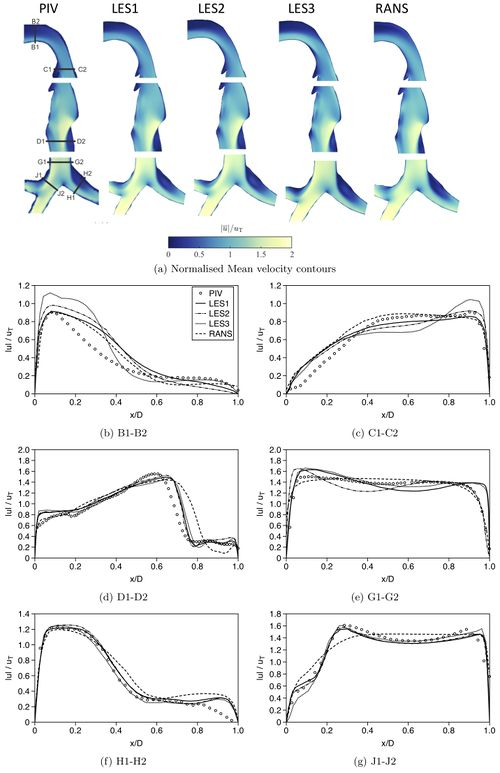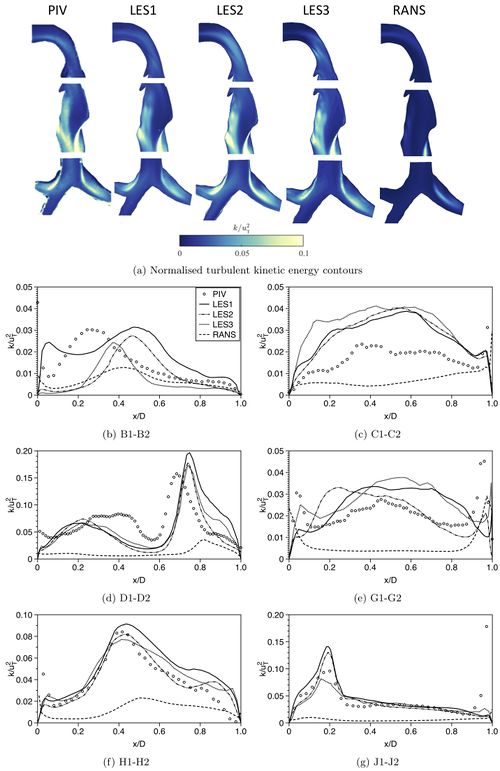# Airflow in the human upper airways

## Evaluation

Figure 23 shows comparisons of normalised in-plane mean velocity contours and profiles at the locations of the PIV measurement planes IV-VI, between the measurements, LES1-3 and RANS computation using the RNG k-ε model. In PIV, only the in-plane velocity components were measured. Therefore, at planes IV & V (mouth-throat and trachea, see figure 6(b)), the in-plane mean velocity is calculated as:

$|u|={\sqrt {u_{x}^{2}+u_{y}^{2}}}\qquad (8).$At planes VI (main bifurcation and bronchi, see figure 6(b)), the in-plane mean velocity is calculated as:

$|u|={\sqrt {u_{y}^{2}+u_{z}^{2}}}\qquad (9).$The velocity to normalise the simulation results is the bulk speed of air in the trachea at a flowrate of 60L/min, $u_{T}=4.8m/s$. Deviations from the PIV-measured data are evident in the calculations near the inlet of the model, at station B1-B2. These are more pronounced in LES3, in which a uniform velocity profile was prescribed at the inlet, and persist further downstream at station C1-C2. The differences near the inlet (station B1- B2) between the measurements and the results from the rest of the computations (LES1&2 and RANS) are probably associated to the differences in the inlet velocity conditions between the experiment and the simulations. Notable deviations are evident in RANS predicted velocity profile at stations D, H and J (see discussion in section Numerical Accuracy for RANS). At most of the stations, LES predictions for the mean velocity are in reasonable accordance independently of the method used.

Figure 24 shows comparisons of normalised turbulent kinetic energy contours and profiles at the locations of the PIV measurement planes IV-VI, between the measurements, LES and RANS. In PIV, only the in-plane velocity components were measured. Therefore, at planes IV & V (mouth and trachea), the in-plane TKE is calculated as:

$k={\frac {3}{4}}(+)\qquad (10).$At planes VI (main bifurcation and bronchi, see figure 6(b)), the in-plane mean velocity is calculated as:

$k={\frac {3}{4}}(+)\qquad (11).$Compared to RANS, LES perform better in capturing the locations of TKE peaks as well as the TKE levels. Deviations between LES results and measurements are more evident at stations B and C. The differences between LES and the measurements can be attributed to factors such as the different turbulent sampling, inlet conditions and of course errors in both the numerical and experimental parts. RANS consistently underestimate turbulent kinetic energy levels compared to PIV and LES data. This can be explained by the fact that in RANS, the turbulence model must represent a very wide range of scales. In contrast, in LES only the smallest scales are modeled. While the small scales depend only on viscosity, the large ones are affected strongly on boundary conditions. As a result, RANS cannot capture the effect of the large scales of turbulence as good as the LES and tend to underpredict turbulent kinetic energy levels.

In summary, LES show better agreement to experimental data than RANS in both the mean and turbulent flowfields. On the other hand, although RANS predictions for the mean flow are in fair agreement to the measured values, RANS systematically under predict TKE values.Figure 23: Comparison of normalised mean velocity contours and profiles at selected stations - shown in (a) - between PIV, LES and RANS data.Figure 24: Comparison of normalised turbulent kinetic energy contours and profiles at selected stations - shown in fig. 22(a) - between PIV, LES and RANS data.

Contributed by: P. Koullapisa, J. Muelab, O. Lehmkuhlc, F. Lizald, J. Jedelskyd, M. Jichad, T. Jankee, K. Bauere, M. Sommerfeldf, S. C. Kassinosa —
aDepartment of Mechanical and Manufacturing Engineering, University of Cyprus, Nicosia, Cyprus
bHeat and Mass Transfer Technological Centre, Universitat Politècnica de Catalunya, Terrassa, Spain
cBarcelona Supercomputing center, Barcelona, Spain
dFaculty of Mechanical Engineering, Brno University of Technology, Brno, Czech Republic
eInstitute of Mechanics and Fluid Dynamics, TU Bergakademie Freiberg, Freiberg, Germany
fInstitute Process Engineering, Otto von Guericke University, Halle (Saale), Germany